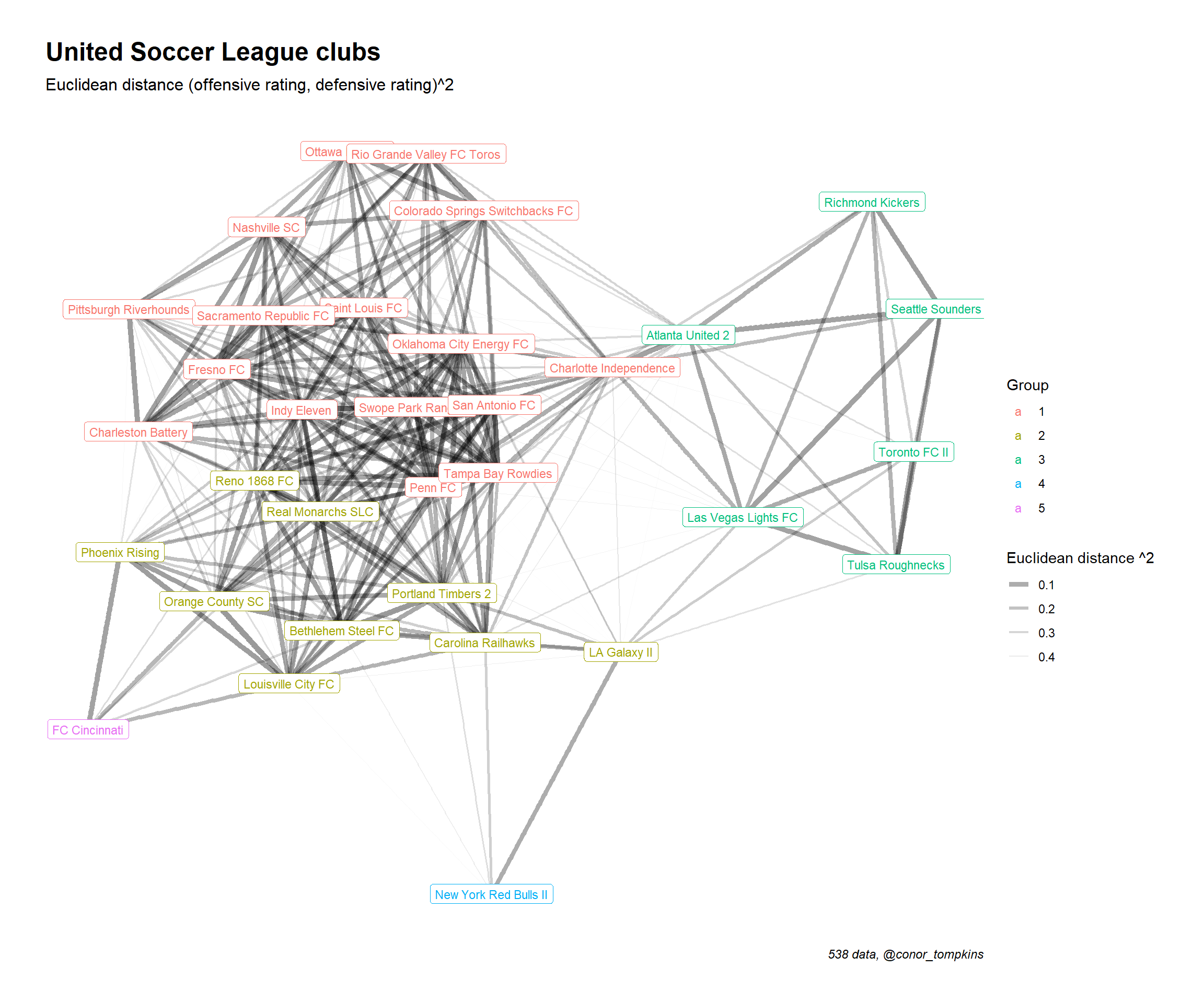Networking USL clubs with Euclidean distance

Euclidean distance is a simple way to measure the distance between two points. It can also be used to measure how similar two sports teams are, given a set of variables. In this post, I use Euclidean distance to calculate the similarity between USL clubs and map that data to a network graph. I will use the 538 Soccer Power Index data to calculate the distance.

Setup

``````library(tidyverse)
library(broom)
library(ggraph)
library(tidygraph)
library(viridis)

set_graph_style()``````

This code downloads the data from 538’s GitHub repo and does some light munging.

``````read_csv("https://projects.fivethirtyeight.com/soccer-api/club/spi_global_rankings.csv", progress = FALSE) %>%
filter(league == "United Soccer League") %>%
mutate(name = str_replace(name, "Arizona United", "Phoenix Rising")) -> df

df``````
``````## # A tibble: 33 x 7
##     rank prev_rank name                league              off   def   spi
##    <int>     <int> <chr>               <chr>             <dbl> <dbl> <dbl>
##  1   255       256 FC Cincinnati       United Soccer Le~  1.49  1.53  45.0
##  2   361       340 Phoenix Rising      United Soccer Le~  1.37  1.72  38.5
##  3   419       396 Louisville City FC  United Soccer Le~  1.28  1.85  34.1
##  4   427       428 Orange County SC    United Soccer Le~  1.23  1.81  33.5
##  5   481       513 Pittsburgh Riverho~ United Soccer Le~  0.77  1.4   29.7
##  6   490       461 Bethlehem Steel FC  United Soccer Le~  1.14  1.97  28.9
##  7   491       460 Real Monarchs SLC   United Soccer Le~  1.04  1.84  28.9
##  8   494       481 New York Red Bulls~ United Soccer Le~  1.5   2.49  28.4
##  9   496       497 Charleston Battery  United Soccer Le~  0.8   1.53  28.1
## 10   499       491 Reno 1868 FC        United Soccer Le~  1.01  1.86  27.8
## # ... with 23 more rows``````

Calculate Euclidean distance

This is the code that measures the distance between the clubs. It uses the 538 offensive and defensive ratings.

``````df %>%
select(name, off, def) %>%
column_to_rownames(var = "name") -> df_dist

#df_dist
#rownames(df_dist) %>%

df_dist <- dist(df_dist, "euclidean", upper = FALSE)

df_dist %>%
tidy() %>%
arrange(desc(distance)) -> df_dist

#df_dist %>%
#  count(item1, sort = TRUE) %>%
#  ggplot(aes(item1, n)) +
#  geom_point() +
#  coord_flip() +
#  theme_bw()``````

Network graph

In this snippet I set a threshhold for how similar clubs need to be to warrant a connection. Then I graph it using tidygraph and ggraph. Teams that are closer together on the graph are more similar. Darker and thicker lines indicate higher similarity.

``````distance_filter <- .5

df_dist %>%
mutate(distance = distance^2) %>%
filter(distance <= distance_filter) %>%
as_tbl_graph() %>%
mutate(community = as.factor(group_edge_betweenness())) %>%
ggraph(layout = "kk", maxiter = 1000) +
geom_edge_fan(aes(edge_alpha = distance, edge_width = distance)) +
geom_node_label(aes(label = name, color = community), size = 3) +
scale_color_discrete("Group") +
scale_edge_alpha_continuous("Euclidean distance ^2", range = c(.4, 0)) +
scale_edge_width_continuous("Euclidean distance ^2", range = c(2, 0)) +
labs(title = "United Soccer League clubs",
subtitle = "Euclidean distance (offensive rating, defensive rating)^2",
x = NULL,
y = NULL,
caption = "538 data, @conor_tompkins")``````